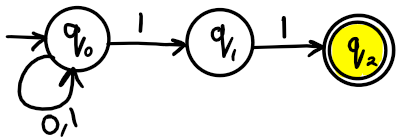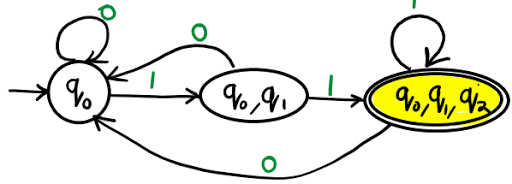# Equivalent of DFA and NFA

RGPV 2010, 02
Q. Write short note on equivalent of DFA and NDFA ?

Ans.
Every DFA is an NDFA.
If from a regular set an NDFA is created than there may be chances of existence of DFA.
DFA is 5 tuple machine:
M = (Q, Σ, δ, q0, F)

1. Q is a finite non empty set of states.
2. Σ is a finite non empty set of input symbols.
3. δ is a transition function, QXΣ int to Q
4. q0 is an initial state belong to Q.
5. F is the set of final states belong to Q.

NDFA is 5 tuple machine:

1. M = ( Q, Σ, δ, q0, F )
2. Q is a finite non empty set of states.
3. Σ is a finite non empty set of input symbols.
4. δ is a transition function, QXΣ int to 2Q
5. q0 is an initial state belong to Q.
6. F is the set of final states belong to Q.

Problem 01: Convert the following Non-Deterministic Finite Automata (NDFA) to Deterministic Finite Automata (DFA).Transition table for NDFA from above NDFA transition diagram

 State Input 0 Input 1 ->q0 q0 q0, q1 q1 – *q2 q2 – –

Transition table for DFA from above NDFA transition table

 State Input a Input b ->q0 q0 {q0, q1} {q0, q1} q0 *{q0, q1, q2} *{q0, q1, q2} q0 *{q0, q1, q2}

Transition diagram from above DFA transition tableReference:

1. Introduction to Automata Theory Language & Computation, Hopcroft& Ullman,
2. Theory of Computation, Chandrasekhar & Mishra, PHI.